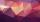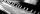# Quotient

Determine the quotient and the second member of the geometric progression where a3=10, a1+a2=-1,6 a1-a2=2,4.

Result

q =  -5
a2 =  -2

#### Solution:Leave us a comment of example and its solution (i.e. if it is still somewhat unclear...):

Showing 0 comments:Be the first to comment!## Next similar examples:

1. Geometric progression 2There is geometric sequence with a1=5.7 and quotient q=-2.5. Calculate a17.
2. Q of GPCalculate quotient of geometric progression if a1=5 a a1+a2=12.
3. GP - 8 itemsDetermine the first eight members of a geometric progression if a9=512, q=2
4. Five membersWrite first 5 members geometric sequence and determine whether it is increasing or decreasing: a1 = 3 q = -2
5. CoefficientDetermine the coefficient of this sequence: 7.2; 2.4; 0.8
6. Tenth memberCalculate the tenth member of geometric sequence when given: a1=1/2 and q=2
7. GP membersThe geometric sequence has 10 members. The last two members are 2 and -1. Which member is -1/16?
8. Geometric sequence 4It is given geometric sequence a3 = 7 and a12 = 3. Calculate s23 (= sum of the first 23 members of the sequence).
9. Six termsFind the first six terms of the sequence a1 = -3, an = 2 * an-1
10. PianoIf Suzan practicing 10 minutes at Monday; every other day she wants to practice 2 times as much as the previous day, how many hours and minutes will have to practice on Friday?
11. Holidays - on poolChildren's tickets to the swimming pool stands x € for an adult is € 2 more expensive. There was m children in the swimming pool and adults three times less. How many euros make treasurer for pool entry?
12. Theorem proveWe want to prove the sentense: If the natural number n is divisible by six, then n is divisible by three. From what assumption we started?
13. PowersExpress the expression ? as the n-th power of the base 10.
14. Expression with powersIf x-1/x=5, find the value of x4+1/x4
15. PowerNumber ?. Find the value of x.
16. AlgebraX+y=5, find xy (find the product of x and y if x+y = 5)
17. One halfOne half of ? is: ?